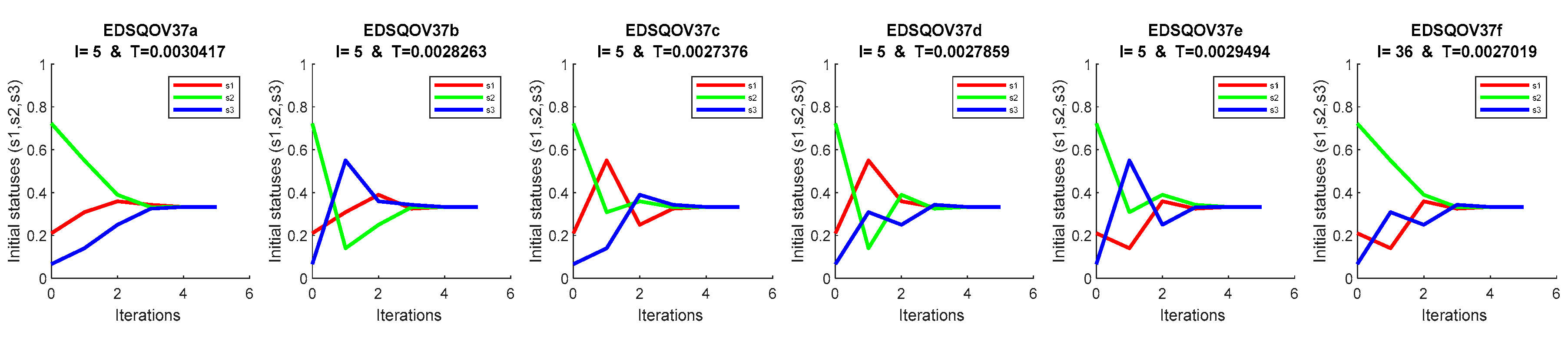Next Article in Journal
Previous Article in Journal
Symmetric Fuzzy Stochastic Differential Equations with Generalized Global Lipschitz Condition
Article

# EDSQ Operator on 2DS and Limit Behavior

1
Computational Intelligence Research Group, Faculty of Information and Communication Technology, International Islamic University Malaysia, Kuala Lumpur 53100, Malaysia
2
King Abdulaziz City for Science and Technology (KACST), Riyadh 12354, Saudi Arabia
3
Department of Information Technology, College of Computer Sciences and Information Technology College, Majmaah University, Al-Majmaah 11952, Saudi Arabia
4
Faculty of Information and Communication Technology, International Islamic University Malaysia, Kuala Lumpur 53100, Malaysia
5
Department of Computer Science, Community College, King Saud University, Riyadh 11437, Saudi Arabia
6
Department of Health Informatics, College of Public Health and Health Informatics, Qassim University, Qassim 51452, Saudi Arabia
7
Department of Natural and Applied Sciences, Faculty of Community College, Majmaah University, Majmaah 11952, Saudi Arabia
*
Authors to whom correspondence should be addressed.
Symmetry 2020, 12(5), 820; https://doi.org/10.3390/sym12050820
Received: 16 April 2020 / Revised: 4 May 2020 / Accepted: 12 May 2020 / Published: 16 May 2020

## Abstract

This paper evaluates the limit behavior for symmetry interactions networks of set points for nonlinear mathematical models. Nonlinear mathematical models are being increasingly applied to most software and engineering machines. That is because the nonlinear mathematical models have proven to be more efficient in processing and producing results. The greatest challenge facing researchers is to build a new nonlinear model that can be applied to different applications. Quadratic stochastic operators (QSO) constitute such a model that has become the focus of interest and is expected to be applicable in many biological and technical applications. In fact, several QSO classes have been investigated based on certain conditions that can also be applied in other applications such as the Extreme Doubly Stochastic Quadratic Operator (EDSQO). This paper studies the behavior limitations of the existing 222 EDSQ operators on two-dimensional simplex (2DS). The created simulation graph shows the limit behavior for each operator. This limit behavior on 2DS can be classified into convergent, periodic, and fixed.
Keywords:

## 1. Introduction

Discrete dynamical systems have been a subject of interest in many scientific and engineering research applications. The theoretical framework of such systems has been presented in [1,2,3,4,5] using stochastic analysis for population genetics. These dynamical systems consist of set point networks that require symmetrical interactions [6,7]. The work has then been extended in  by deploying the majorization technique to derive new notions and properties of the related systems.
A specific case based on linear dynamic systems has been used in  to address the agreement issue in the technology science and engineering areas, and has subsequently been reformulated to a nonlinear dynamic system for the same problem [10,11]. In effect, the focus on applications-related studies has shifted to nonlinear dynamical systems in order to address such potential problems. The main reason for this shift is merited by their advantage of being more efficient than their linear counterparts. The quadratic stochastic operator (QSO) constitutes one particular class of nonlinear dynamic systems of particular interest, as introduced in , along with its related research history. As a matter of fact, many classes of QSO have been established for the application of consensus [13,14,15,16,17,18,19,20]. This class has been extensively examined and investigated in various works yielding many sub-classes in the process. These QSO sub-classes have been thoroughly investigated as volterra [21,22], dissipative [23,24], Doubly Stochastic Quadratic Operator (DSQOs), and Extreme Doubly Stochastic Quadratic Operator (EDSQOs) . The limit conduct study constitutes the major issue for most nonlinear dynamic models . The mapping of such finite dimensional simplex in this case is what defines the QSO . Lyubich has studied the limit conduct of QSO on one-dimensional simplex (1DS) in , albeit with the exception of the high dimensional simplex. Other studies have introduced additional properties for certain QSO sub-classes that are deemed more significant than others . One of these classes is the DSQO.
The points of DSQO are presented in the form of a polyhedron in dimensional space. This polyhedron of DSQO will consist of extreme points which are called EDSQO. Since DSQO constitutes a sub-class of QSO, the QSO is DSQO if the operator of $s$ is majorized by , where $V s$ is the operator (output), $" ≺ "$ signifies majorization, and $s$ is the value of inputs . Consensually, the values of $s$ is arranged in a non-increasing sequence . Mirsky  identified the necessary conditions for special stochastic and doubly stochastic matrices. In addition, an overview of the concept of the doubly stochastic matrix along with earlier open problems were addressed in . Ryff  extended the related work by introducing the notion of the majorization theory in regard to the convex hull for some functions of doubly stochastic transformations. Moreover, a clear illustration on the significance of these operators and the underlying conceptual perception of majorization were presented in , while the theory and necessary conditions for DSQO were described in . Since the dimensional space can be found in finite sets, studying the limit is expected to be rather complicated . Due to this reason, the study on the EDSQOs’ limit behavior has been examined in specific dimensions, in particular from the two-dimensional simplex (2DS) point of view, where such operators are defined using majorization techniques. Such studies have proved the existence of 37 extreme points based on the permutation of the DSQO sets on 2DS. Therefore, it can be used to form 222 EDSQOs on 2DS, as shown in . However, the existing studies have failed to simulate the limit of all 222 EDSQOs on 2DS. Hence, our work is designed to study the limit of these 222 EDSQOs on 2DS and generalize the outcomes for finite-dimensional simplex.
Many previous studies have considered complex non-linear models for synchronization to a complex network. The issues of these models have to do with synchronizing and convergence, as well as in their complex calculations. For example, the Duffiny oscillator  involves complex, nonlinear, dynamic equations that convert to convergence and synchronization. However, the downside of this model that it requires highly complicated computations in the form of second-order differential equations as compared to the EDSQ model, which is a more flexible nonlinear model that can deal with a large number of nodes for the networks.
This work presents the limit of all 222 EDSQ operators on 2DS. The paper consists of an introductory part (Section 1), methodology (Section 2), the theoretical result (Section 3), and the discussion and numerical solution (Section 4), followed by the research conclusion (Section 5).

## 2. Methods

It is appropriate to start this discussion by introducing the concept of majorization and its rules for DSQO operators.
Definition 1:
QSO is a nonlinear dynamical model defined on finite dimensional simplex$( m − 1 )$ [14,22,31]:
Definition 2:
The set offalls within simplex if$s i > 0$. The set consists of${ 0 , … , 1 , … , 0 }$and is called a set of extreme points, while a simplex center set consists of${ 1 m , 1 m , 1 m }$.
Definition 3:
The nonlinear model of QSO is defined as :
where $p i j , k$ is a transaction matrix under conditions [22,32]:
The QSO has applications in population genetics, where the population is defined as $m$ species. Here, is defined as independent members of the population in their initial states. The communication from member $i t h$ to member $j t h$ called ’parents’ allows the transition to produce a new member $k$. This communication among the members configures a transition matrix $P i j , k$ and produces an inherited factor. Each individual member has a separate transition matrix eventually consisting of the total of these separated matrices with the sum of all its elements being equal to 1. This means that the transition matrix is denoted by $P i j , k$ which has distributed to $m$ matrices $P i j , k = ( p i j , 1 | p i j , 2 | … | p i j , m )$, where $p i j , m$ is symmetric and nonnegative.
In this case, a steady state of the potential parents xx appears $s i s j$ .
Definition 4:
The matrix$P i j , k$is doubly stochastic if:
Definition 5:
The operator$V : S m − 1$is called a doubly stochastic operator (DSQO) if:
The set of elements ) in DSQO are re-arranged in a non-increasing order , then:
From this point, the majorization concept stipulates that $y$ majorizes $s$, which is denoted by $y ≻ s$ or $s$ is majorized by $y$. The doubly stochastic matrix $P$ has been defined according to  stating that if $y = P s$ then $y ≻ s$. It follows that in DSQO all $s$ elements belong to the simplex $P s ≻ s$ if $P$ is a doubly stochastic matrix.
Therefore, the particular operator of $V ( s )$ is called DSQO if the transition matrix $P$ is doubly stochastic in accordance with the majorization theory .
In other words, the operator of $V ( s )$ is defined a DSQO if the transition matrix $P i j , k$ in Equation (2) satisfies the condition $V ( s ) ≺ s$, which means:
where $α$ is a subblock in the matrix that contains elements $( m − 1 )$ and its sum should be $≤ ( m − 1 )$ .
Definition 6:
As mentioned above, DSQO has vertices points which are called extremes, which is why we call it EDSQO. The additional conditions for DSQO to become EDSQO [15,32] are that the transition matrix should be:
a.
b.
Definition 7:
To define the limit behavior of EDSQO we should first insert the initial values ofto render it$V ( s 0 )$, while$V ( s 0 )$is inserted into$V 2 ( s 0 )$, and so on. The series ofis termed the limit of the trajectory of the operator$V ( s 0 )$. Therefore, the QSO, DSQO, and EDSQO can be called the evolutionary process, where$s 0$is the initial generation and$V n + 1 ( s 0 )$the next generation. To study the limit for the operator we find the limit as$n → ∞$until the trajectory reaches the fixed points. Since the set of points are fixed on$( 1 m , 1 m , 1 m )$, the set has converged on the center of the simplex. These sequences of generations are also called iterations.
Definition 8:
If the transition matrix of an individual member$s d$has interactions only with itself$s d d$and with itself and others$s d k$, then the interaction here is called a selfish interaction.
Definition 9:
If the transition matrix of an individual member$s d$has at least an interaction between other members$s k l$, then the interaction is called an unselfish interaction.
Definition 10:
If the trajectory of the set points does not change through the limit of$n → ∞$, then the set points are fixed. Even if the set points start from different values and are fixed after several iterations, the set of points are called fixed where $V i ( x 0 ) = x 0$,for all$i = n → ∞$.
Definition 11:
If the set points of the trajectory have exchanged among themselves through the limit of$n → ∞$, without changing the values, then the set points are called periodic where$V i ( s 0 ) = s 0$,for all$i = n → ∞$.

## 3. Theoretical Result

The limit behavior of EDSQO has been studied theoretically in this section.
Theorem 1:
Considering DSQO as an evaluation operator$V$that has been defined on 2DS, there exists a limit of each operator of DSQO on 2DS.
Proof:
Suppose that $V ( n ) ( s m )$ is EDSQO on 2DS where $n$ is the number of iterations and $m$ is the number of set points, then series of $V ( n ) ( s m )$ is = . Based on the majorization concept for DSQO that $s ≺ V ( s )$, it means that:
$V ( 0 ) ( s m ) ≻ V ( 1 ) ( s m ) ≻ V ( 2 ) ( s m ) ≻ … ≻ V ( n ) ( s m )$
In a non-increasing re-arrangement for $s m$, we obtain:
$V ( n ) ( s 1 ) ≤ … ≤ V ( 2 ) ( s 1 ) ≤ V ( 1 ) ( s 1 ) < V ( 0 ) ( s 1 )$
Therefore, the maximum of the set points increases, while the minimum of the set points decreases monotonically through the series of $V ( n ) ( s m )$ and becomes fixed.
This means that $l i m n → ∞ ∑ i = 1 m V ( n ) ( s m )$ exists.
We can thus conclude that the limit of EDSQO on 2DS exists.

## 4. Discussion and Numerical Solution

In this section, we present the simulation results for all operators of EDSQO on 2DS using the MATLAB software.
First, we outline all 37 EDSQOs on 2DS as follows:
where are EDSQOs names, and each of the 37 EDSQOs will have $3 !$ permuted operators. For instance, for operator $V 1$,
where are the names of $3 !$ permuted EDSQOs. Consequently, we generate $3 ! ∗ 37 = 222$ EDSQOs. These 222 distributed permuted matrices and operators of 37 EDSQOs have been figures on Appendix A.
The limit behavior of each EDSQO’s permutation is as follows (see Figure 1, Figure 2, Figure 3, Figure 4, Figure 5, Figure 6, Figure 7, Figure 8, Figure 9, Figure 10, Figure 11, Figure 12 and Figure 13):
In this section, we explain the Figure 1, Figure 2, Figure 3, Figure 4, Figure 5, Figure 6, Figure 7, Figure 8, Figure 9, Figure 10, Figure 11, Figure 12 and Figure 13. The initial statuses $( s 1 , s 2 , s 3 )$ are random, $I$ is the number of iterations for convergence, and $T$ is the computation time to converge. Each figure is assigned a name under EDSQO, followed by the number of operators from , where each operator from is given one row of Figures which are the permutations of 3!.
From the simulation result it can be observed that are altogether 222 EDSQOs, whereby 198 EDSQOs always converge to the average $( 1 3 )$ or the center (see Figure 1, Figure 2, Figure 3, Figure 4, Figure 5, Figure 6, Figure 7, Figure 8, Figure 9, Figure 10, Figure 11, Figure 12 and Figure 13). In the case of 18 EDSQOs , it was found that each has one fixed point and two converged points $( s i 0 + s i 0 2 )$ (see Figure 2, Figure 3 and Figure 4, Figure 7, Figure 8, Figure 9 and Figure 10, Figure 12). Moreover, five EDSQOs $( V 28 a , V 28 b , V 28 c , V 28 d , V 28 e )$ had periodic points (see Figure 10), and one EDSQO had only fixed points (see Figure 10).
This simulation analysis proves that the limit behavior of DSQOs in the form of converged or periodic or fixed points. That is due to the fact that if the EDSQO has positive points and none of them has a selfish interaction, these points converge (the 198 EDSQOs). However, if one of these points has a selfish interaction (the 18 EDSQOs and $( V 28 f )$), they do not change and are fixed. Consequently, if there are two or more points that have selfish communications, they are periodized (the five EDSQOs).
In conclusion, the study of the limit behavior of all DSQOs on finite dimensional simplex is considerably complex. This is due to the fact that the set points of DSQOs represent a relatively large class. Thus, we have taken a subclass of DSQO that can be studied more conveniently and be used to create a general theory for the entire DSQO class. Therefore, the study has been confined to the EDSQOs class on 2DS due to its low-complexity computation. The limit behavior of EDSQOs on 2DS has been determined and the analysis result has been generalized to the DSQO class.

## 5. Conclusion and Future Work

We have attempted to analyze a considerably challenging issue related to the nonlinear mathematical model. We have deliberated the trajectories limit behavior of all extreme points for DSQO on 2DS. Subsequently, we have generalized the implications of the result of those EDSQO trajectories’ limit behavior by applying them to the whole class of DSQO. In the process, our work has demonstrated the existence of the EDSQOs’ limit behavior. Through simulation, we have been able to observe the set points’ convergence to the center if they have positive statuses and unselfish communications or their fixation if they have positive statuses and selfish communications or their periodization if they have two or more points with selfish communications. For any future work on this subject, we recommend the study of EDSQ operators on infinite dimensional simplex. Furthermore, it is recommended to evaluate the model for certain specific applications and compare it with existing similar models for the consensus problem.

## Author Contributions

Conceptualization, Data curation, methodology, analysis, writing, supervision, R.A.; project administration, Resources, Writing—review and editing, H.A.; validation, Writing—review and editing, B.A.; validation, Review, S.W.; validation, Writing—review and editing, A.A.; supervision, resources, S.S.A.; resources, S.A. All authors have read and agreed to the published version of the manuscript.

## Funding

This research was funded by Majmaah University under grant number [RGP-2019-29].

## Acknowledgments

The first author would like to thank the Faculty of Information and Communication Technology, International Islamic University Malaysia. Additionally, the authors extend their appreciation to the Deanship of Scientific Research at Majmaah University for funding this work under project number NO (RGP-2019-29).

## Conflicts of Interest

The authors declare no conflict of interest.

## Appendix A. The EDSQOs on 2DS

The permutation probabilities distribution 3! for each distribution of the 37 matrices and operators $V i$ of EDSQOs on 2DS are 222 where $i$ the name of matrices and operators from 1 to 37, while are the factorial of 3 for each matrix and operator as follows: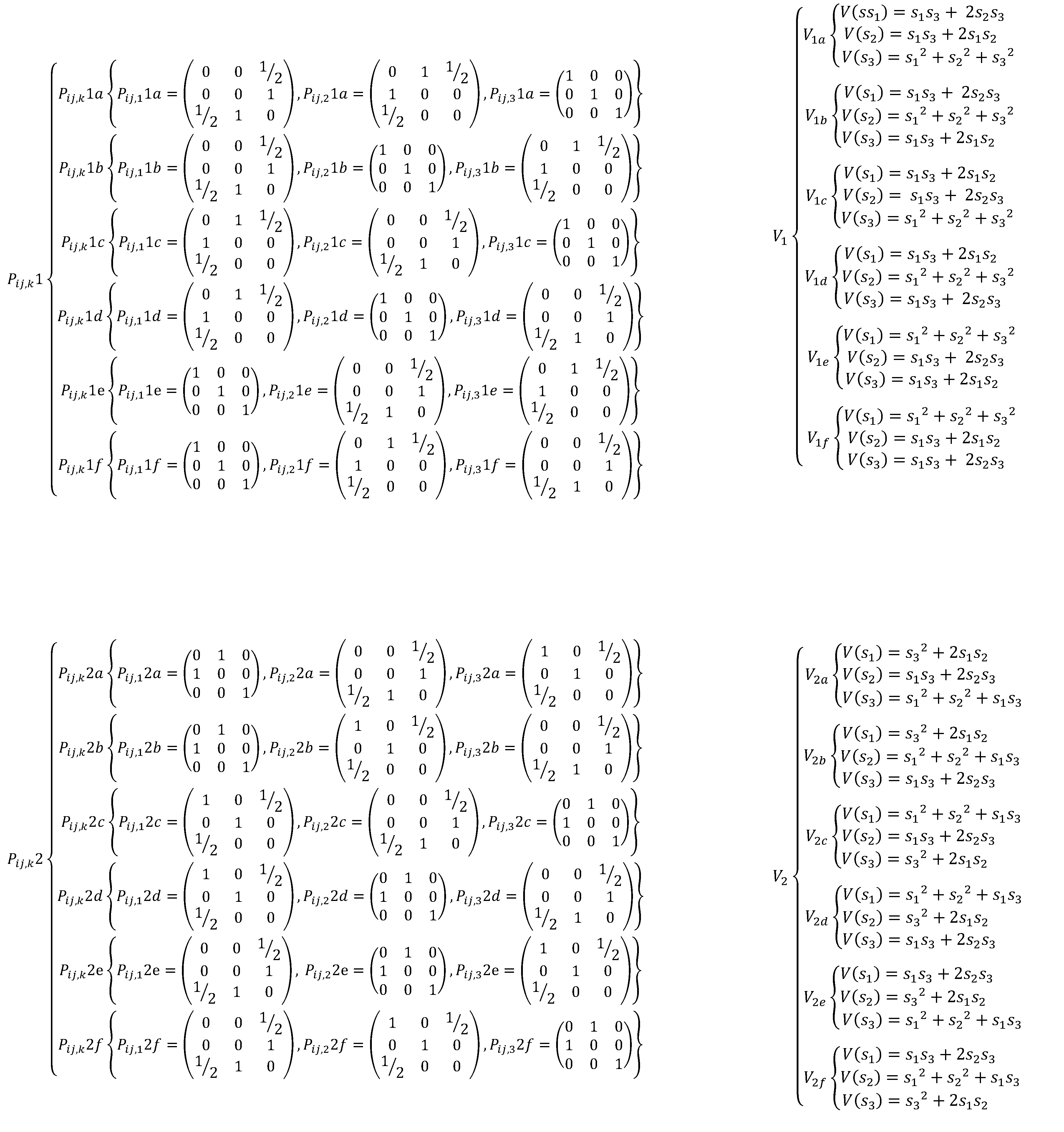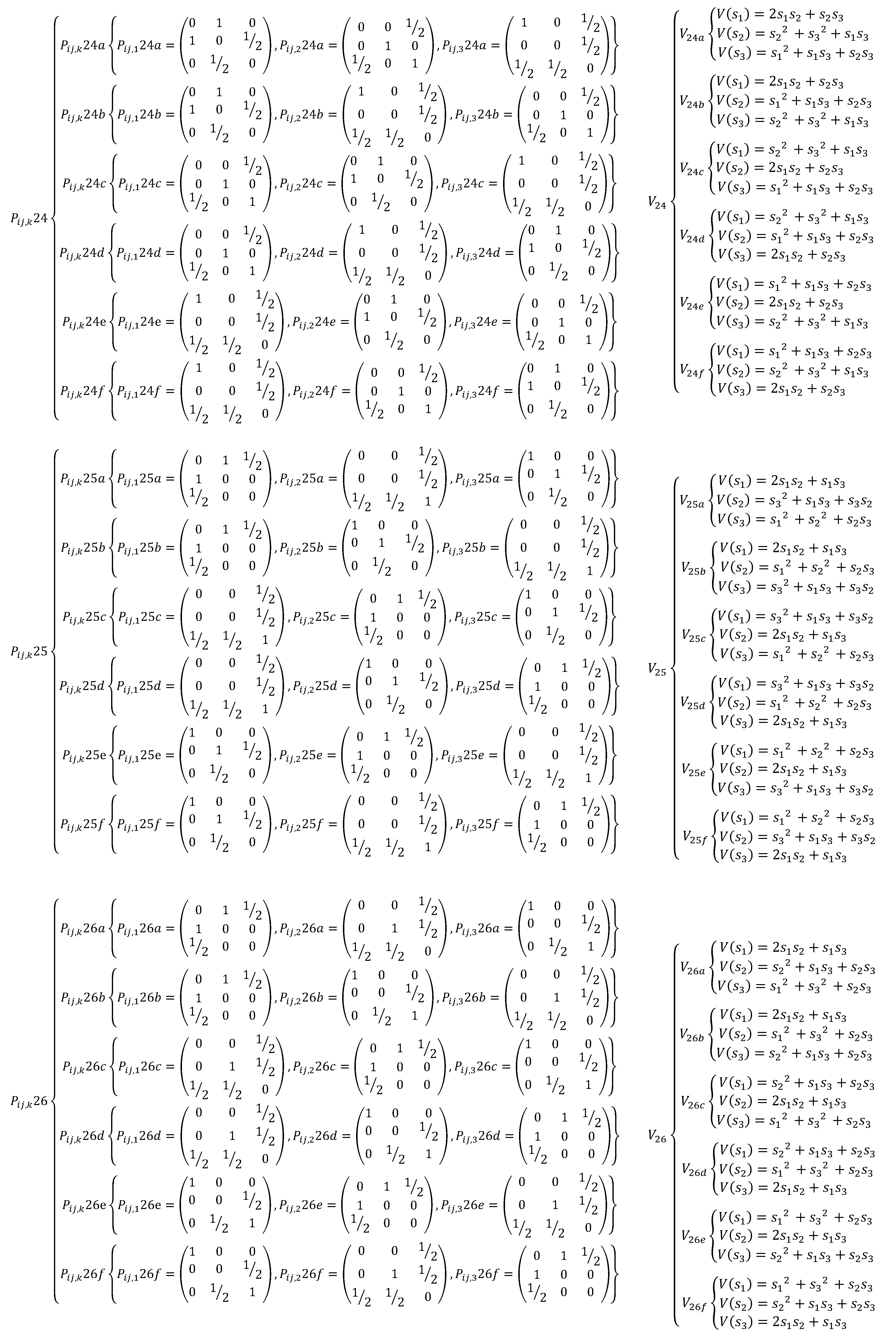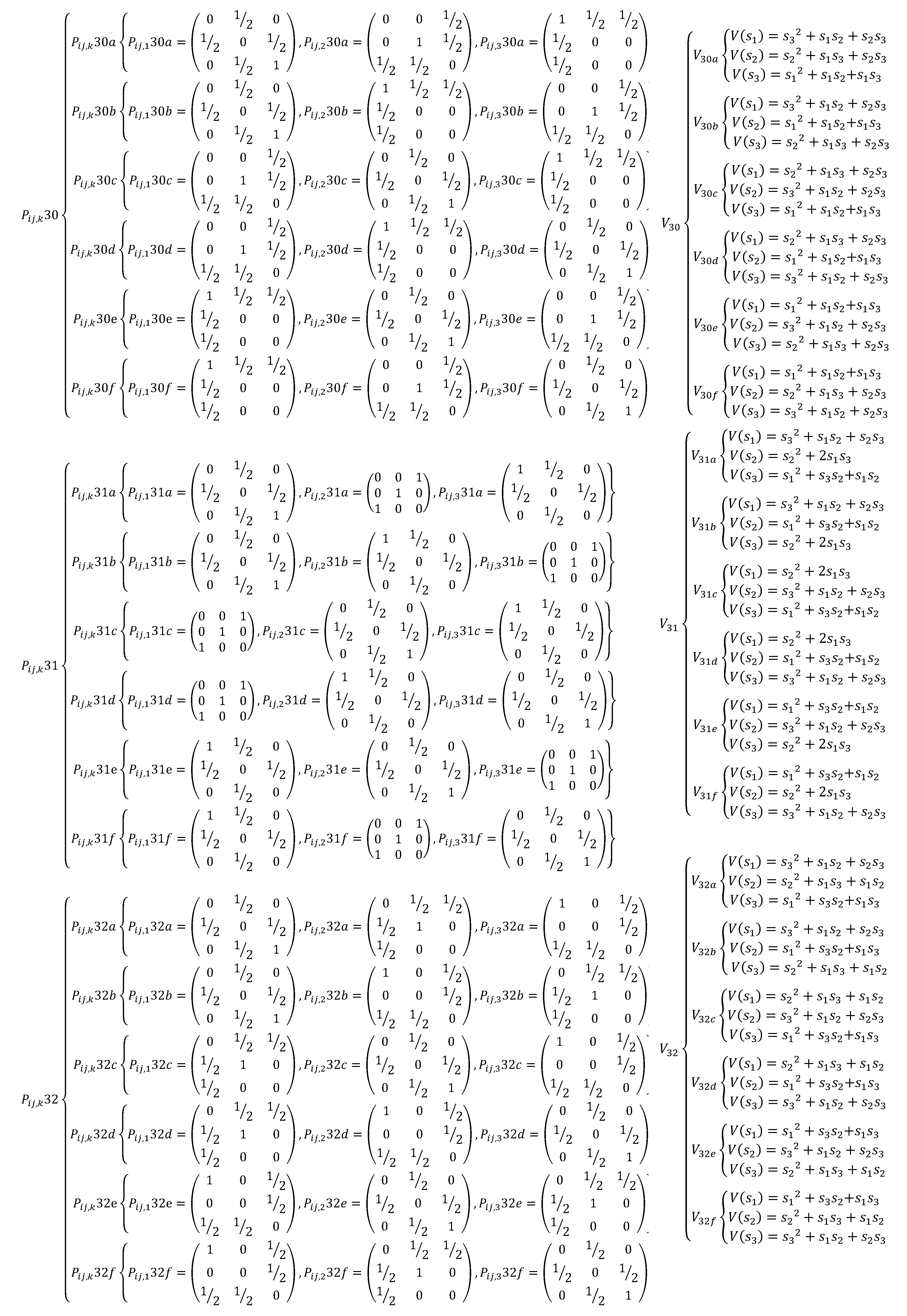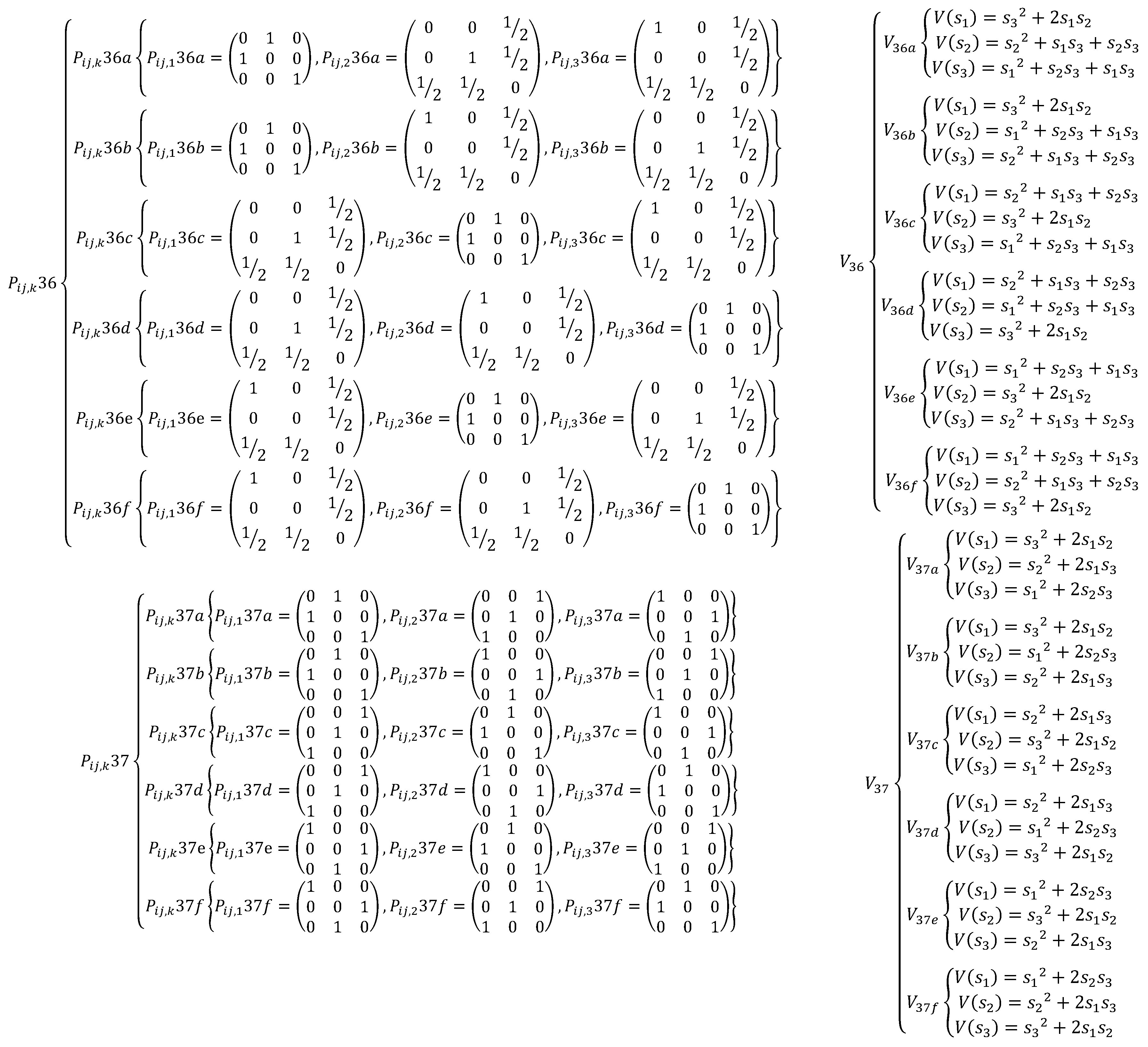## References

1. Lyubich, Y.I.; Vulis, D.; Karpov, A.; Akin, E. Mathematical structures in population genetics. Biomathematics 1992, 22, 373. [Google Scholar]
2. Abdulghafor, R.; Shahidi, F.; Zeki, A.; Turaev, S. Dynamics classifications of extreme doubly stochastic quadratic operators on 2d simplex. In Advanced Computer and Communication Engineering Technology; Springer: Berlin/Heidelberg, Germany, 2016; pp. 323–335. [Google Scholar]
3. Abdulghafor, R.; Shahidi, F.; Zeki, A.; Turaev, S. Dynamics of doubly stochastic quadratic operators on a finite-dimensional simplex. Open Math. 2016, 14, 509–519. [Google Scholar] [CrossRef]
4. Shahidi, F.; Ganikhodzhaev, R.; Abdulghafor, R. The dynamics of some extreme doubly stochastic quadratic operators. Middle East J. Sci. Res. 2013, 13, 59–63. [Google Scholar]
5. Abdulghafor, R.; Turaev, S.; Abubakar, A.; Zeki, A. The extreme doubly stochastic quadratic operators on two dimensional simplex. In Proceedings of the 2015 4th International Conference on Advanced Computer Science Applications and Technologies (ACSAT), Kuala Lumpur, Malaysia, 8–10 December 2015; pp. 192–197. [Google Scholar]
6. Abdulghafor, R.; Abdullah, S.S.; Turaev, S.; Hassan, R. The nonlinear limit control of EDSQOs on finite dimensional simplex. Automatika 2019, 60, 404–412. [Google Scholar] [CrossRef]
7. Abdulghafor, R.; Turaev, S.; Zeki, A. Necessary and Sufficient Conditions for Complementary Stochastic Quadratic Operators of Finite-Dimensional Simplex. Sukkur IBA J. Comput. Math. Sci. 2017, 1, 22–27. [Google Scholar] [CrossRef]
8. Ganikhodzhaev, R.N. On the definition of bistochastic quadratic operators. Russ. Math. Surv. 1993, 48, 244–246. [Google Scholar] [CrossRef]
9. DeGroot, M.H. Reaching a consensus. J. Am. Stat. Assoc. 1974, 69, 118–121. [Google Scholar] [CrossRef]
10. Abdulghafor, R.; Turaev, S.; Zeki, A.; Shahidi, F. The convergence consensus of multi-agent systems controlled via doubly stochastic quadratic operators. In Proceedings of the 2015 International symposium on agents, multi-agent systems and robotics (ISAMSR), Putrajaya, Malaysia, 18–19 August 2015; pp. 59–64. [Google Scholar]
11. Abdulghafor, R.; Turaev, S.; Tamrin, I. Nonlinear consensus for multi-agent systems using positive intractions of doubly stochastic quadratic operators. Int. J. Perceptive Cogn. Comput. 2016, 2, 19–22. [Google Scholar] [CrossRef]
12. Bernstein, S. Solution of a mathematical problem connected with the theory of heredity. Ann. Math. Stat. 1942, 13, 53–61. [Google Scholar] [CrossRef]
13. Abdulghafor, R.; Almotairi, S.; Almohamedh, H.; Turaev, S.; Almutairi, B. Nonlinear Consensus Protocol Modified from Doubly Stochastic Quadratic Operators in Networks of Dynamic Agents. Symmetry 2019, 11, 1519. [Google Scholar] [CrossRef]
14. Abdulghafor, R.; Abdullah, S.S.; Turaev, S.; Zeki, A.; Al-Shaikhli, I. Linear and nonlinear stochastic distribution for consensus problem in multi-agent systems. Neural Comput. Appl. 2018, 32, 261–277. [Google Scholar]
15. Abdulghafor, R.; Abdullah, S.S.; Turaev, S.; Othman, M. An overview of the consensus problem in the control of multi-agent systems. Automatika 2018, 59, 143–157. [Google Scholar] [CrossRef]
16. Abdulghafor, R.; Almotairi, S.; Almohamedh, H.; Almutairi, B.; Bajahzar, A.; Almutairi, S.S. A Nonlinear Convergence Consensus: Extreme Doubly Stochastic Quadratic Operators for Multi-Agent Systems. Symmetry 2020, 12, 1519. [Google Scholar] [CrossRef]
17. Abdulghafor, R.; Turaev, S. Consensus of fractional nonlinear dynamics stochastic operators for multi-agent systems. Inf. Fusion 2018, 44, 1–21. [Google Scholar] [CrossRef]
18. Abdulghafor, R.; Turaev, S.; Izzuddin, M. Nonlinear Models for Distributed Consensus Modified from DSQO in Networks of Dynamic Agents. In Proceedings of the 4th International Conference on Mathematical Sciences, Putrajaya, Malaysia, 15–17 November 2016. [Google Scholar]
19. Abdulghafor, R.; Turaev, S.; Zeki, A.; Al-Shaikhli, I. Reach a nonlinear consensus for MAS via doubly stochastic quadratic operators. Int. J. Control 2018, 91, 1431–1459. [Google Scholar] [CrossRef]
20. Abdulghafor, R.; Turaev, S.; Zeki, A.; Abubaker, A. Nonlinear convergence algorithm: Structural properties with doubly stochastic quadratic operators for multi-agent systems. J. Artif. Intell. Soft Comput. Res. 2018, 8, 49–61. [Google Scholar] [CrossRef]
21. Ganikhodzhaev, R.N. Quadratic stochastic operators, Lyapunov functions, and tournaments. Russ. Acad. Sci. Sb. Math. 1993, 76, 489. [Google Scholar] [CrossRef]
22. Ganikhodzhaev, R.; Shahidi, F. Doubly stochastic quadratic operators and Birkhoff’s problem. Linear Algebra Appl. 2010, 432, 24–35. [Google Scholar] [CrossRef]
23. Shahidi, F.A.; Osman, M.T.A. The Limit behavior of the trajectories of dissipative quadratic stochastic operators on finite-dimensional simplex. J. Differ. Equations Appl. 2013, 19, 357–371. [Google Scholar] [CrossRef]
24. Shahidi, F. On dissipative quadratic stochastic operators. Appl. Math. Inf. Sci. 2008, 2, 211–223. [Google Scholar]
25. Shahidi, F. Necessary and sufficient conditions for doubly stochasticity of infinite-dimensional quadratic operators. Linear Algebra Appl. 2013, 438, 96–110. [Google Scholar] [CrossRef]
26. Mirsky, L. Even doubly-stochastic matrices. Math. Ann. 1961, 144, 418–421. [Google Scholar] [CrossRef]
27. Mirsky, L. Results and Problems in the Theory of Doubly-Stochastic Matrices. Group 1963, 334, 319–334. [Google Scholar] [CrossRef]
28. Ryff, J.V. On the representation of doubly stochastic operators. Pacific J. Math 1963, 13, 1379–1389. [Google Scholar] [CrossRef]
29. Ryff, J.V. Orbits of L1 Functions under Doubly Stochastic Transformations. Trans. Am. Math. Soc. JSTOR 1965, 117, 92–100. [Google Scholar]
30. Wang, Z.; Duan, Z.; Cao, J. Impulsive synchronization of coupled dynamical networks with nonidentical Duffing oscillators and coupling delays. Chaos Interdiscip. J. Nonlinear Sci. 2012, 22, 13140. [Google Scholar] [CrossRef]
31. Olkin, I.; Marshall, A.W. Inequalities: Theory of Majorization and Its Applications; Academic Press: Cambridge, MA, USA, 2016; Volume 143. [Google Scholar]
32. Ganikhodzhaev, R.N.; Rozikov, U.A. Quadratic stochastic operators: Results and open problems. arXiv 2009, arXiv:0902.4207. [Google Scholar] [CrossRef]
Figure 1. Limit behavior of permuted operators of V1, V2, and V3 for Extreme Doubly Stochastic Quadratic Operator (EDSQO) on two-dimensional simplex (2DS).
Figure 1. Limit behavior of permuted operators of V1, V2, and V3 for Extreme Doubly Stochastic Quadratic Operator (EDSQO) on two-dimensional simplex (2DS).Figure 2. Limit behavior of permuted operators of V4, V5, and V6 for EDSQO on 2DS.
Figure 2. Limit behavior of permuted operators of V4, V5, and V6 for EDSQO on 2DS.Figure 3. Limit behavior of permuted operators of V7, V8, and V9 for EDSQO on 2DS.
Figure 3. Limit behavior of permuted operators of V7, V8, and V9 for EDSQO on 2DS.Figure 4. Limit behavior of permuted operators of V10, V11, and V12 for EDSQO on 2DS.
Figure 4. Limit behavior of permuted operators of V10, V11, and V12 for EDSQO on 2DS.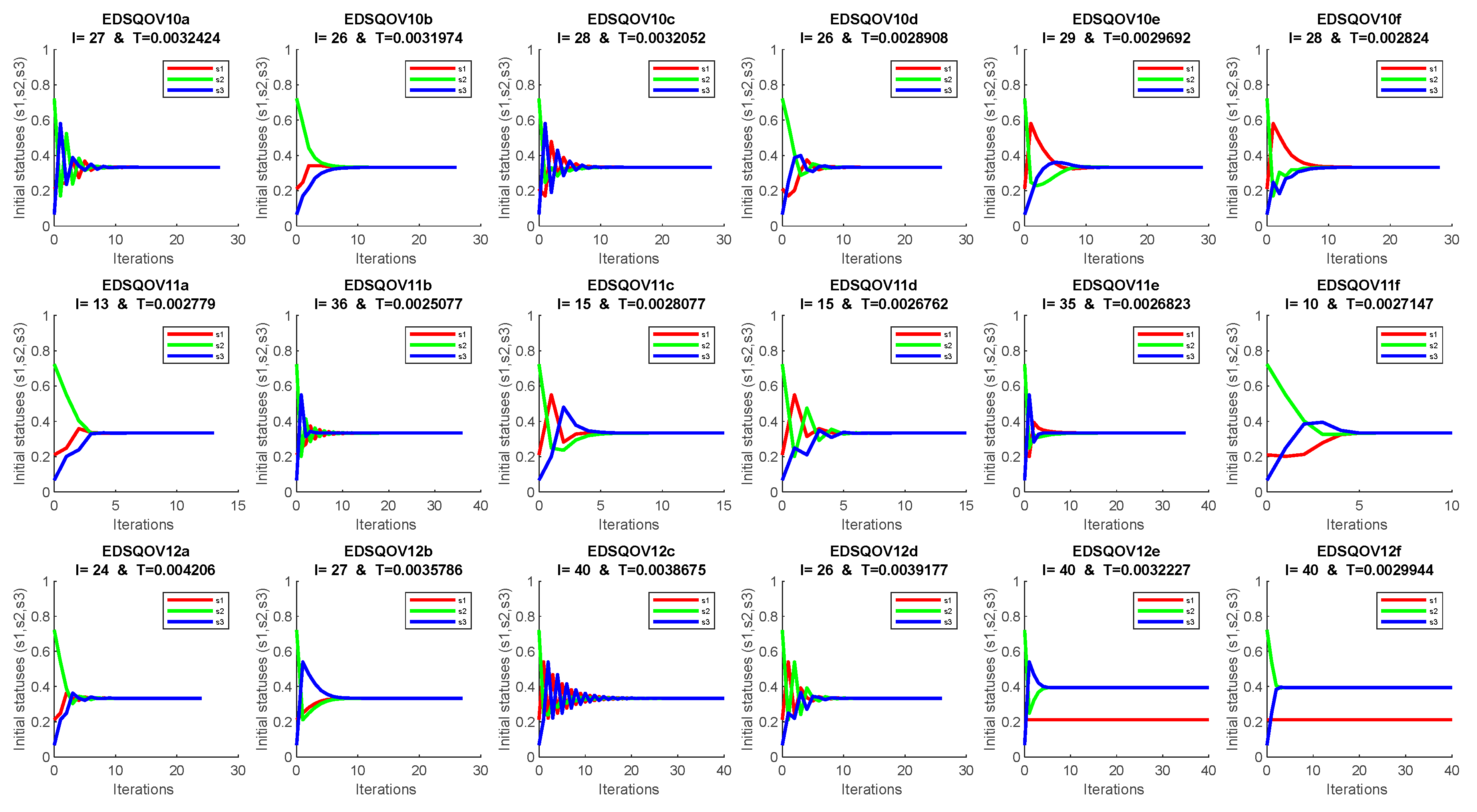Figure 5. Limit behavior of permuted operators of V13, V14, and V15 for EDSQO on 2DS.
Figure 5. Limit behavior of permuted operators of V13, V14, and V15 for EDSQO on 2DS.Figure 6. Limit behavior of permuted operators of V16, V17, and V18 for EDSQO on 2DS.
Figure 6. Limit behavior of permuted operators of V16, V17, and V18 for EDSQO on 2DS.Figure 7. Limit behavior of permuted operators of V19, V20, and V21 for EDSQO on 2DS.
Figure 7. Limit behavior of permuted operators of V19, V20, and V21 for EDSQO on 2DS.Figure 8. Limit behavior of permuted operators of V22, V23, and V24 for EDSQO on 2DS.
Figure 8. Limit behavior of permuted operators of V22, V23, and V24 for EDSQO on 2DS.Figure 9. Limit behavior of permuted operators of V25, V26, and V27 for EDSQO on 2DS.
Figure 9. Limit behavior of permuted operators of V25, V26, and V27 for EDSQO on 2DS.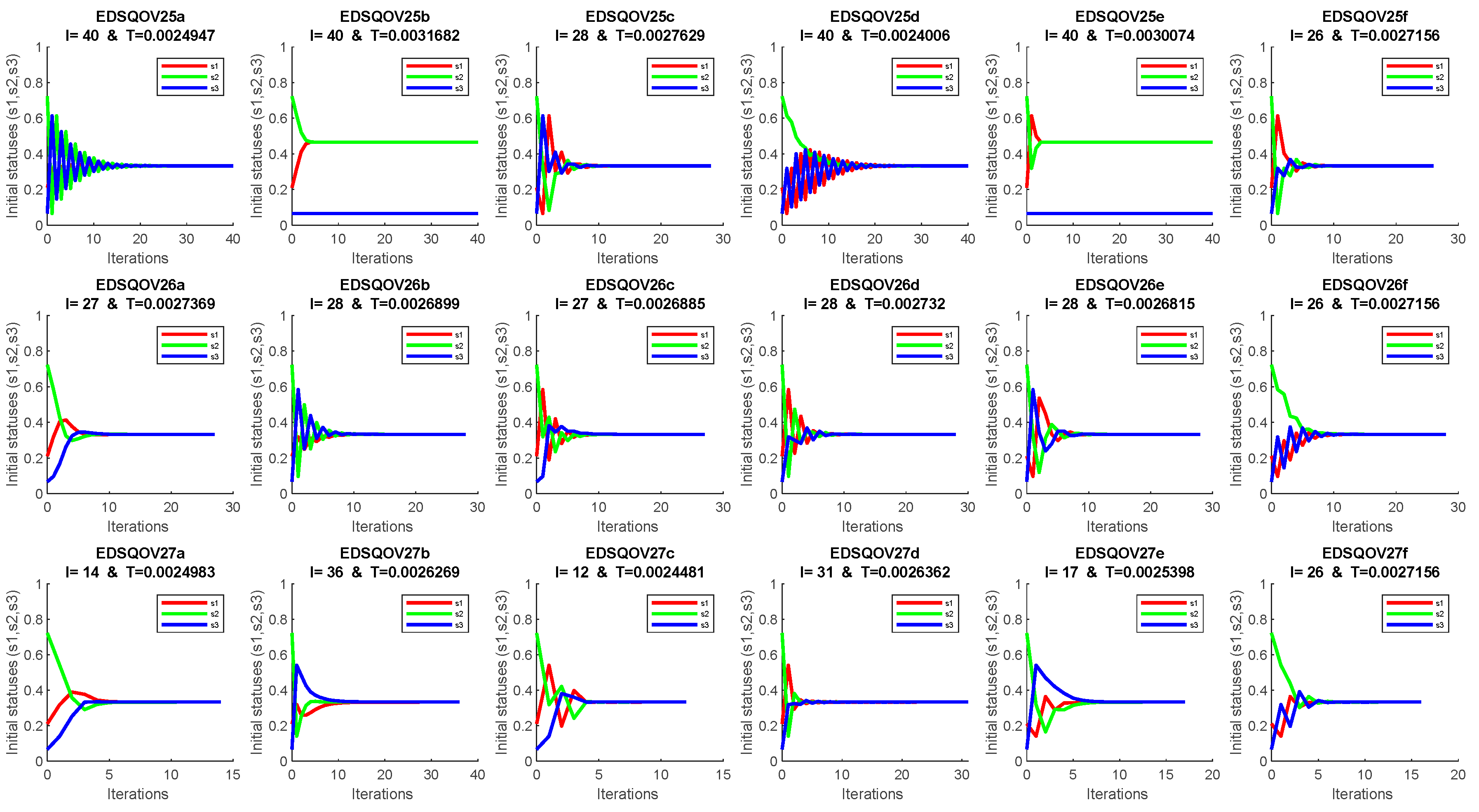Figure 10. Limit behavior of permuted operators of V28, V29, and V30 for EDSQO on 2DS.
Figure 10. Limit behavior of permuted operators of V28, V29, and V30 for EDSQO on 2DS.Figure 11. Limit behavior of permuted operators of V31, V32, and V33 for EDSQO on 2DS.
Figure 11. Limit behavior of permuted operators of V31, V32, and V33 for EDSQO on 2DS.Figure 12. Limit behavior of permuted operators of V34, V35, and V36 for EDSQO on 2DS.
Figure 12. Limit behavior of permuted operators of V34, V35, and V36 for EDSQO on 2DS.Figure 13. Limit behavior of permuted operators of V37 for EDSQO on 2DS.
Figure 13. Limit behavior of permuted operators of V37 for EDSQO on 2DS.